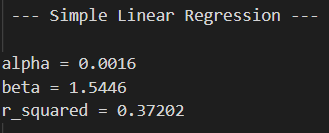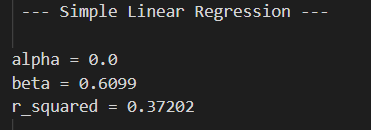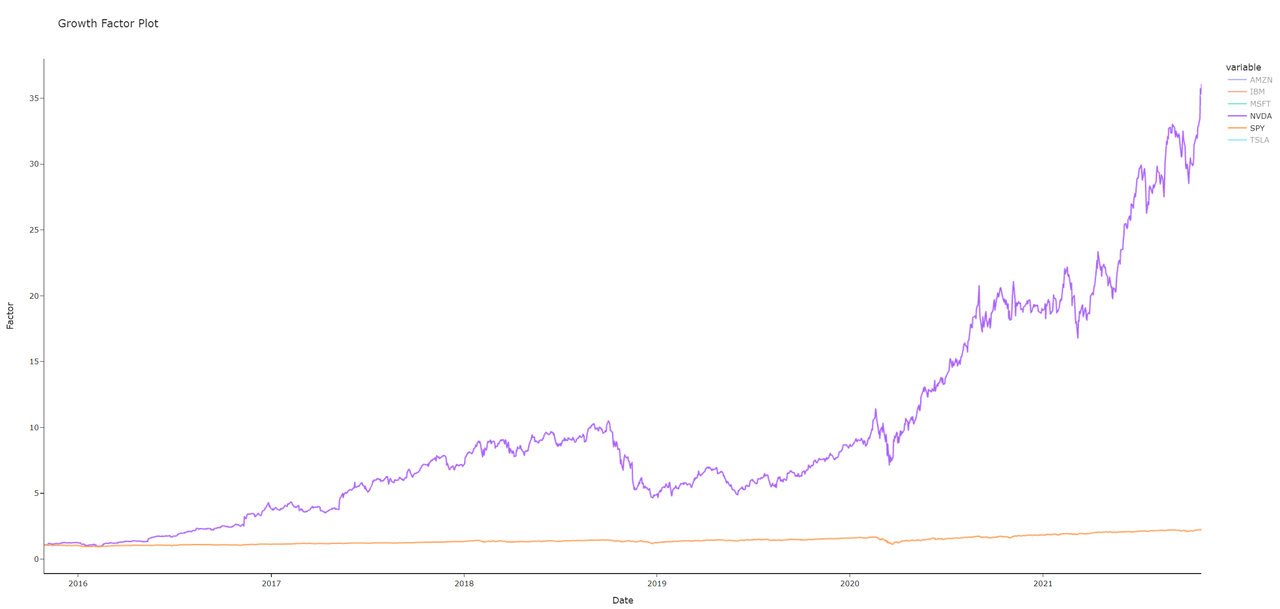Explaining results

Anything related to deep learning, machine learning, data science, and artificial intelligence. Course topic requests can go here.
greek_quant
Posts: 2
Joined: Mon Nov 22, 2021 10:18 pm

Explaining results

Hi LazyProgrammer (LP),

Hi everyone,

LP thanks for the great courses you deliver to us. I would deem them concise, precise and the best place to get some food for thought and further research on any of the topics.

Now I have a question mainly coming from standardizing inputs before say, performing a simple linear regression. I will use my own examples.

I performed two runs of a simple linear regression using the returns of SPY and NVDA. NVDA is dependent variable (y) while the market benchmark - SPY - is the independent one(x). One run using just the return series of each asset and another run standardizing each series by subtracting its mean and dividing by the standard deviation.

I get the following outputs. The first one is without standardization and the second is with standardization.So from above we can see that when standardizing we lose some info around the alpha (outperformance) of NVDA while the sensitivity i.e. beta is also shrunk to 0.61 implying that in general when SPY moves by 1 then NVDA moves by 0.61 instead of around 1.5 from the beta of the regression without standardization.

Given that we can look at the growth factor for the two time series, we can clearly provide evidence that the small alpha coefficient is important in approximating changes but what is more important is the beta.Is this an example where some experience is needed to derive the conclusion of whether to use standardization or not? If I had to report these results somewhere then I would need to possibly explain the 0.61 beta. Does standardizing inputs also encapsulates some extra important things to keep in mind (domain knowledge)?

A bit lengthy but hope the message is clearly conveyed. Let me know if I am mumbling heregreek_quant
Posts: 2
Joined: Mon Nov 22, 2021 10:18 pm

Re: Explaining results

****** UPDATE ******

Found it...I should be a bit more patient the next time before I post something.

https://en.wikipedia.org/wiki/Standardized_coefficient